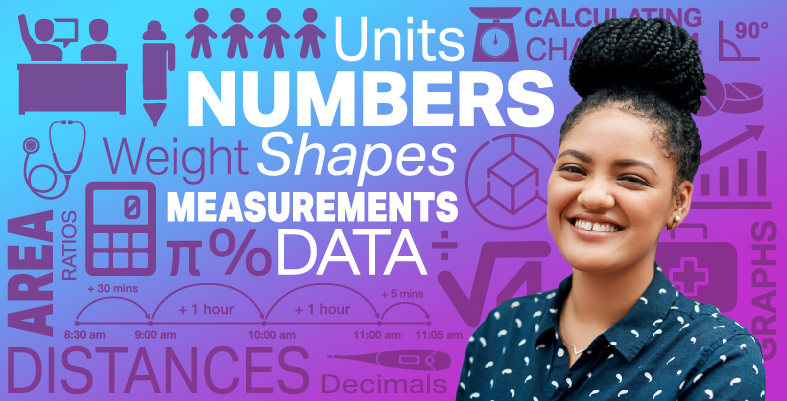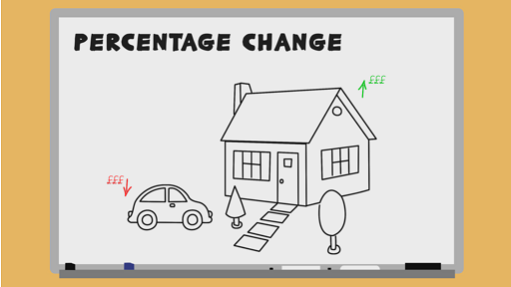Everyday maths for Health and Social Care and Education Support 2

Start this free course now. Just create an account and sign in. Enrol and complete the course for a free statement of participation or digital badge if available.

Free course

# 5.2 Percentage change

Watch the video below on how to calculate percentage change, then complete Activity 10.Skip transcript

#### Transcript

You will already be familiar with the concept that if you buy a new car, when you come to sell it, its value is likely to have decreased. On the other hand, if you're lucky, when you buy a house and then wish to sell, you may be able to sell it for more than you bought it for.

The percentage difference between the original price and the sale price is called percentage change. This can be useful for working out the percentage profit, or loss, or finding out by what percentage an item has changed in value. It's important to recognise that percentage change can be either positive, if the price has increased, or negative, if the value of the item has gone down.

To calculate the percentage change, we need to use the simple formula: percentage change equals the difference, divided by the original, multiplied by 100. 'Difference' refers to the difference between the two values. That is, the cost before and after. 'Original' means the original value of the item.

Let's use this formula in an example. A train ticket used to cost £24. The price has gone up to £27.60. How do we find the percentage increase? First, you need to find the difference in cost. The difference = £27.60 - £24, which is £3.60.

Next, divide this difference by the original cost, and then multiply by 100. £3.60 divided by £24, x 100, = 15. The cost of the train ticket has increased by 15%.

Now try another example. You bought a car for £4,500 and you managed to sell it for £3,200. What is the percentage decrease? You should give your answer to two decimal places. The difference is £4,500 - £3,200, which = £1,300. Divide this by the original cost of £4,500. 1,300 divided by 4,500, x 100, equals a 28.89% per cent decrease. Now practise using the percentage change formula in the next activity.

End transcript

Interactive feature not available in single page view (see it in standard view).

## Activity 10: Percentage change formula

Practise using the percentage change formula which you learned about in the video above on the three questions below. Where rounding is required, give your answer to two decimal places.

1. Last year the cost of sending a child to after school club every day of the school year was £1,300. This year the cost has risen to £1,450. What is the percentage increase?

1. Difference: £1,450 − £1,300 = £150

• a.Original: £1,300

• b.Percentage change = £150 ÷ £1,300 × 100 = 11.54% increase (rounded to two d.p.)

1. You bought your house 10 years ago for £155,000. You are able to sell your house for £180,000. What is the percentage increase the house has made?

1. Difference: £180,000 − £155,000 = £25,000

• a.Original: £155,000

• b.Percentage change = £25,000 ÷ £155,000 × 100 = 16.13% increase (rounded to two d.p.)

1. You purchased your car 3 years ago for £4,200. You sell it to a buyer for £3,600. What is the percentage decrease of the car?

1. Difference: £4,200 − £3,600 = £600

• a.Original: £4200

• b.Percentage change = £600 ÷ £4,200 × 100 = 14.29% decrease (rounded to two d.p.)

FSM_SSH_2